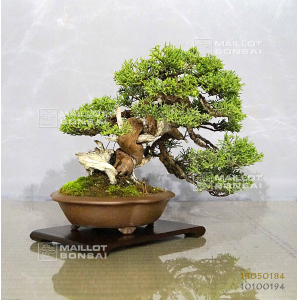##### The Japanese Bonsai specialist
Direct order Contact Help / Services Newsletter# Juniperus chinensis itoigawa ref 18050184

Juniperus chinensis itoigawaref. : 10296

2.400,00

Available quantity : 1Order

###### Description

Height of the tree without its pot: 190 mm. Width of the branches:: 260 mm. Chinese artisanal quality pot: 150*40 mm in a lotus flower form.

Remarkable bonsaï for collector. Moyogi style.

Strong trunk of about 40/50 mm.

Juniper with graceful trays, a shari on its twisted trunk and jins. Itoigawa variety with green canopy thin scales, particuartly vigorous and perfectly adapted to the bonsaï cultivation. Contrary to the classic juniperus chinensis, this variety only loses little foliage in the beginning of summer.

The bark of this tree is formed because it is more than 30/35 years old.

Not any strong wire or cutting wound.

This tree was imported from Master Tomoya Nishikawa, who have formed it from cutting in 35 years and have always repoted it every 2 years in the same pot size!

It is a personal selection of Master Tomoya Nishikawa of itoigawa junipers with particularly short scales.

The repoting was done in April 2018, you will have to do it again in April 2021.

Admire the knotted trunk, that makes that bonsaï unique.

The whitening with jin product of the dead trunks, softly toned with charcoal will give it a beautiful ash color.

Do you already have this complete book on juniper?

Shelf non-included. Photographed in October 2019.

#bonsai 5.6 #with 5.4 #itoigawa 4.8 #this 4.4 #have 4.4 #juniperus 3.9 #chinensis 3.9 #years 3.5 #trunk 3.5 #tree 3.4

Formule
(( ROUND((CHAR_LENGTH(b.article_nom)-CHAR_LENGTH(REPLACE(b.article_nom, 'with', '')))/LENGTH('with')) + ROUND((CHAR_LENGTH(b.article_description)-CHAR_LENGTH(REPLACE(b.article_description, 'with', '')))/LENGTH('with')) ) * 5.4) + (( ROUND((CHAR_LENGTH(b.article_nom)-CHAR_LENGTH(REPLACE(b.article_nom, 'itoigawa', '')))/LENGTH('itoigawa')) + ROUND((CHAR_LENGTH(b.article_description)-CHAR_LENGTH(REPLACE(b.article_description, 'itoigawa', '')))/LENGTH('itoigawa')) ) * 4.8) + (( ROUND((CHAR_LENGTH(b.article_nom)-CHAR_LENGTH(REPLACE(b.article_nom, 'have', '')))/LENGTH('have')) + ROUND((CHAR_LENGTH(b.article_description)-CHAR_LENGTH(REPLACE(b.article_description, 'have', '')))/LENGTH('have')) ) * 4.4) + (( ROUND((CHAR_LENGTH(b.article_nom)-CHAR_LENGTH(REPLACE(b.article_nom, 'this', '')))/LENGTH('this')) + ROUND((CHAR_LENGTH(b.article_description)-CHAR_LENGTH(REPLACE(b.article_description, 'this', '')))/LENGTH('this')) ) * 4.4) + (( ROUND((CHAR_LENGTH(b.article_nom)-CHAR_LENGTH(REPLACE(b.article_nom, 'chinensis', '')))/LENGTH('chinensis')) + ROUND((CHAR_LENGTH(b.article_description)-CHAR_LENGTH(REPLACE(b.article_description, 'chinensis', '')))/LENGTH('chinensis')) ) * 3.9) + (( ROUND((CHAR_LENGTH(b.article_nom)-CHAR_LENGTH(REPLACE(b.article_nom, 'juniperus', '')))/LENGTH('juniperus')) + ROUND((CHAR_LENGTH(b.article_description)-CHAR_LENGTH(REPLACE(b.article_description, 'juniperus', '')))/LENGTH('juniperus')) ) * 3.9) + (( ROUND((CHAR_LENGTH(b.article_nom)-CHAR_LENGTH(REPLACE(b.article_nom, 'bonsai', '')))/LENGTH('bonsai')) + ROUND((CHAR_LENGTH(b.article_description)-CHAR_LENGTH(REPLACE(b.article_description, 'bonsai', '')))/LENGTH('bonsai')) ) * 3.6) + (( ROUND((CHAR_LENGTH(b.article_nom)-CHAR_LENGTH(REPLACE(b.article_nom, 'trunk', '')))/LENGTH('trunk')) + ROUND((CHAR_LENGTH(b.article_description)-CHAR_LENGTH(REPLACE(b.article_description, 'trunk', '')))/LENGTH('trunk')) ) * 3.5) + (( ROUND((CHAR_LENGTH(b.article_nom)-CHAR_LENGTH(REPLACE(b.article_nom, 'years', '')))/LENGTH('years')) + ROUND((CHAR_LENGTH(b.article_description)-CHAR_LENGTH(REPLACE(b.article_description, 'years', '')))/LENGTH('years')) ) * 3.5) + (( ROUND((CHAR_LENGTH(b.article_nom)-CHAR_LENGTH(REPLACE(b.article_nom, 'tree', '')))/LENGTH('tree')) + ROUND((CHAR_LENGTH(b.article_description)-CHAR_LENGTH(REPLACE(b.article_description, 'tree', '')))/LENGTH('tree')) ) * 3.4)

## Secure payment## Delivery

Our logistic partners :